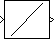# Constant Ramp

Generate ramp signal with length based on input dimensions

• Library:
• DSP System Toolbox / Signal Operations

•## Description

The Constant Ramp block generates the constant ramp signal

`y = (0:L-1)*m + b `

where m is the slope specified by the scalar Slope parameter, and b is the y-intercept specified by the scalar Offset parameter.

For an unoriented vector input, L is equal to the length of the input vector. For an N-D input array, the length L of the output ramp is equal to the length of the input in the dimension specified by the Ramp length equals number of or Dimension parameter. The output, y, is always an unoriented vector.

## Ports

### Input

expand all

Input signal used to generate ramp signal, specified as a scalar, vector, matrix, or N-D array.

Data Types: `single` | `double` | `int8` | `int16` | `int32` | `int64` | `uint8` | `uint16` | `uint32` | `uint64` | `Boolean` | `fixed point`

### Output

expand all

Output signal, specified as an unoriented vector. The block determines the length of the output based on the length of the input signal, and the Ramp length equals number of or Dimension parameter.

Data Types: `single` | `double` | `int8` | `int16` | `int32` | `int64` | `uint8` | `uint16` | `uint32` | `uint64` | `Boolean` | `fixed point`

## Parameters

expand all

### Main

Specify whether the length of the output ramp is the number of rows, number of columns, or the length of the specified dimension of the input.

Specify the one-based dimension of the input array that determines the length of the output ramp as a positive integer scalar.

#### Dependencies

To enable this parameter, set Ramp length equals number of to ```Elements in specified dimension```.

Specify the slope of the ramp as a real-valued double-precision scalar.

Specify the scalar y-intercept of the ramp as a real-valued double-precision scalar.

### Data Types

Specify the output data type for this block. You can select one of the following:

• A rule that inherits a data type, for example, `Inherit: Same as input`.

• A built-in data type, such as `double`

• An expression that evaluates to a valid data type, for example, `fixdt(1,16)`

For help setting data type parameters, display the Data Type Assistant by clicking the button.

## Block Characteristics

 Data Types `double` | `fixed point` | `integer` | `single` Direct Feedthrough `no` Multidimensional Signals `no` Variable-Size Signals `no` Zero-Crossing Detection `no`

## Version History

Introduced before R2006a